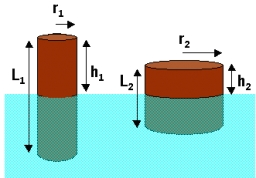# Calculate 19443

Calculate the height of the cylinder when r = 10 mm and S = 800 mm2.
Calculate the radius / r / of the cylinder when the height is 20 mm and S = 1000 mm2.

h1 =  2.7324 mm
r2 =  6.0983 mm

### Step-by-step explanation:Did you find an error or inaccuracy? Feel free to write us. Thank you!

Tips for related online calculators
Are you looking for help with calculating roots of a quadratic equation?

#### Grade of the word problem:

We encourage you to watch this tutorial video on this math problem: